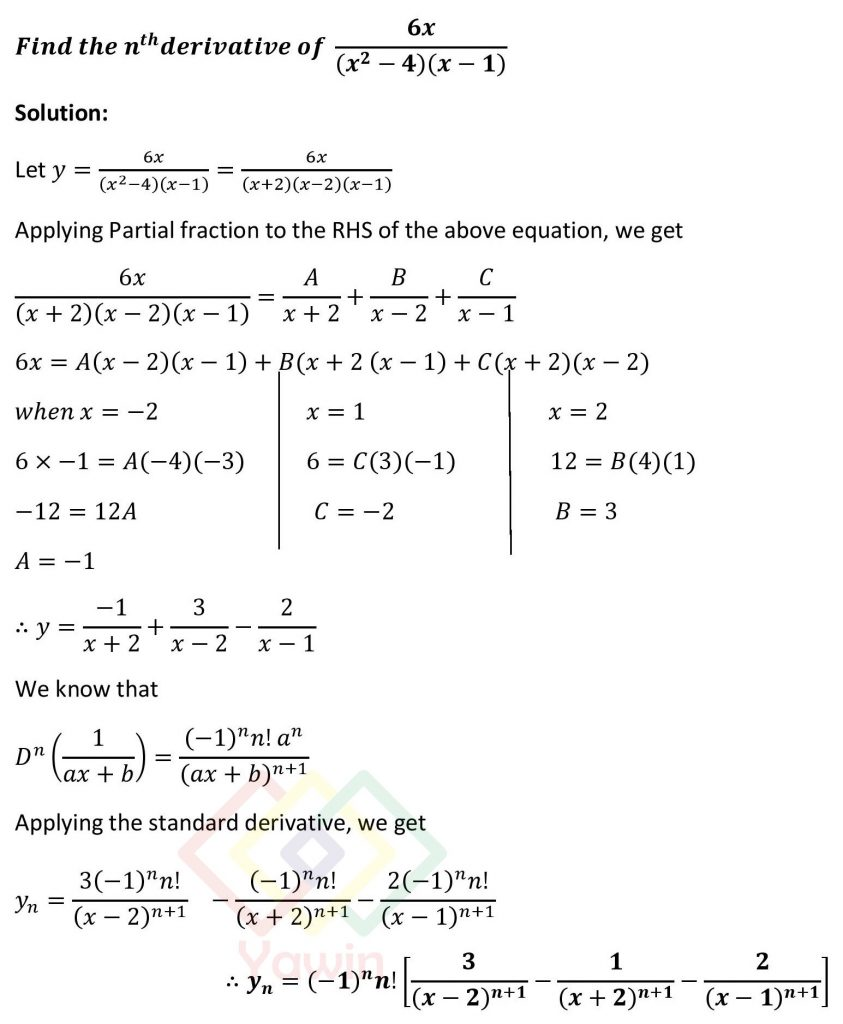Find the nth derivative of 6x/((x^2-4)(x-1))

## Problem## Question

Find the nth derivative of 6x/((x^2-4)(x-1))

nth derivatives